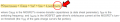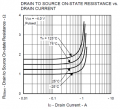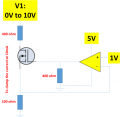# Question on MOSFET specification

#### Tony9168

Joined Mar 6, 2016
32
Hi All,

I am currently doing a project on MOSFET, therefore, i need to understand the specification of the MOSFET in order to make a judgement whether this part can or cannot be used. However, I face some difficulty during the progress, and i need expert advises on the following matter:

Question 1
: How to determine the gate current required for the calculation of the switching loss?
Background 1: I would like to find out the total power dissipation of the MOSFET. However, i am facing the difficulty on calculating the switching loss as i not understand the how to determine the "gate current" of the MOSFET. The confusion come from my understanding toward the operation of the ideal mosfet that there is no current flow to the gate of the mosfet. The drain source resistance is controlled by the gate voltage. Therefore, is the gate current required here referring to the specification "Gate-Source Leakage Current" stated in the datasheet? Please advise.

The document availabe at (page 4): https://pdfserv.maximintegrated.com/en/an/AN1832.pdfQuestion 2: Why the On-resistance getting higher when the drain current higher under the constant gate voltage?
Background 2: I am thinking from the perspective of V=IR. When the voltage is kept constant, shouldn't that the On resistance value should be lower when the drain current increase? This characteristic below is belong to RENESAS, 2SJ648.

https://4donline.ihs.com/images/VipMasterIC/IC/RNCC/RNCCS04513/RNCCS04513-1.pdf?hkey=EF798316E3902B6ED9A73243A3159BB0Question 3:
Why does thermal resistance information is not specify on most of the mosfet datasheet?
Background 3: Need for determination of thermal effect of the mosfet on the actual applicatioin.

#### Jony130

Joined Feb 17, 2009
5,145
e
How to determine the gate current required for the calculation of the switching loss?
The MOSFET gate behaviors just like a capacitor. And this is why at steady state no currenrt will flow.
As first aproximation you can use this Q = I*t--->Ig = Qg*F or I = Qg/T_on .
But in the equation from the application note they want the driver current capability (what current can driver source/sink).

Why the On-resistance getting higher when the drain current higher under the constant gate voltage?
Maybe because at high currents (beyond transistor capabilities) resistance increases with temperature.

Why does thermal resistance information is not specify on most of the mosfet datasheet?
From 2SJ648 we can calculate
Rthja = (Tj- Ta)/Pt = (150°C - 25°C)/0.2W = 625 C°/W

•Tony9168

Joined Mar 10, 2018
3,914
•Tony9168

#### Tony9168

Joined Mar 6, 2016
32
The MOSFET gate behaviors just like a capacitor. And this is why at steady state no currenrt will flow.
As first aproximation you can use this Q = I*t--->Ig = Qg*F or I = Qg/T_on .
But in the equation from the application note they want the driver current capability (what current can driver source/sink).
Thanks for the video. It provide a very clear picture on mosfet operation during switching. The basic idea is to imagine the gate to source as a capacitor, and through the characteristic of the capacitor, it is short initially (current flow) until the capacitor act as open circuit. (no current)

Another i would like to seek for consultation is:

If the application of the mosfet is for current limiting purposes in which the mosfet always operate within its linear region and never cut off, do we need to consider switching loss for the power dissipation?

Joined Mar 10, 2018
3,914
Correct, if its operating small signal there is no switching losses, but there is still dielectric
losses and esr related losses. But fairly small compared to switching loss.

Keep in mind that a C takes in energy, over time (that's power) and then delivers
it back, over time(that's power again), so net in ideal C is zero. But circuit overall,
if it is delivering power to C from non ideal source, like a battery or a smps or a
transformer operated supply, will show power loss each time cap is charged and
discharged.

Regards, Dana.

•Tony9168

#### Jony130

Joined Feb 17, 2009
5,145
If the application of the mosfet is for current limiting purposes in which the mosfet always operate within its linear region and never cut off, do we need to consider switching loss for the power dissipation?
In the "linear region" region? For the MOSFET's the term "linear region" means something else than in BJT's.
In case of a MOSFET's the term "linear region" is used when the MOSFET works as a switch (the MOSFET is fully open).

And when MOSFET work "statically" we always calculate the conduction losses in the MOSFET.

P = Id^2*Rds(on) or P = Id * Vds

•Tony9168

#### Tony9168

Joined Mar 6, 2016
32This is the example of the application of the mosfet in current limiting circuit to clamp the current at max 10mA.

Question 1: Based on the understanding from the reply, to calculate the power loss of the mosfet, i should assumed use the maximum input voltage, V1 = 10V, clamping current =10mA.

Therefore, the ON resistance should be:
V = IR
10V = 10mA x (400 + ON_R + 100)
ON_R = 10V/10mA-500
ON_R = 500 ohm

Power Loss = I2R = (0.01)(0.01)(500) = 0.05W

Question 2: Through my understanding, the current limiting circuit works by regulating the gate voltage of the mosfet by op amps. But, i cant understand how the op amps regulates the gate voltage.

From my point of view, the op amp in the current limiting circuit is acts as an comparator which will produce output voltage either 0V or 5V due to its open loop gain. There is no linear output from the op amp, then, the mosfet should only has ON & OFF state without linear output. Therefore, i unable to understand how the op amp function in this circuit.

Joined Jul 18, 2013
20,084
Last edited:

Joined Mar 10, 2018
3,914
An OpAmp with negative fdbk drives its output such that Vin+ and Vin-
are roughly equal. You can use this basic model and write the equations
and evaluate them as Aol of opamp approaches infinity, eg. take the
limit in below diagram as A approaches infinity.There are tons of resources on web that do the anaysis if you are not
comfortable doing analysis.

So given that Vin+ ~= Vin- you can see that Vref is impressed on the R
that sets current. That means the OpAmp output took on the value of
Vref at its output to set that relationship.

Regards, Dana.

Last edited:

#### Jony130

Joined Feb 17, 2009
5,145
Question 1: Based on the understanding from the reply, to calculate the power loss of the mosfet, i should assumed use the maximum input voltage, V1 = 10V, clamping current =10mA.
In this circuit, the MOSFET will work in the saturation region or in the ohmic region depend on the Vsupply voltage.

Also, you cannot treat the MOSFET as an ordinary resistor when the MOSFET is working in the saturation region.
In saturation, the drain current is directly proportional to the Vgs voltage and Vds voltage has almost no influence on Id current. The MOSFET will behavior as a voltage controlled (Vgs voltage) current source (Id current).

The maximum power dissipation will accrue when Vds = 10V/2 and Id = 10mA ---> Pmax = 5V*10mA = 50mW

https://electronics.stackexchange.com/questions/310613/how-power-dissipation-in-a-transistor-is-less-when-signal-is-present/310691#310691

Question 2: Through my understanding, the current limiting circuit works by regulating the gate voltage of the mosfet by op amps. But, i cant understand how the op amps regulates the gate voltage.
First try to read my old post from here https://forum.allaboutcircuits.com/threads/two-simple-questions-but-im-troubled-by-them.64696/#post-444315 by starting from "The more accurate explanation".

In your circuits, the opamp will work as a voltage follower and the output of the opamp will do whatever it takes to make the voltages at the V"+" input equal to the voltage at V"-" input. The opamp will simply "compare" the V"+" voltage (1V) with the voltage produced by the current flowing through a 100Ω resistor. V"-" = I_load x 100Ω. And if for example, this voltage (the voltage drop across 100Ω) is lower than the voltage at V"+" input, the opamp will turn-on the MOSFET "harder" by increasing the output voltage to make sure that the voltage drop across R3 is equal to the voltage at V"+" input.

Also, do you want to build this circuit in real life?

If so, why did you load the opamp output with such a low resistor value?
What opamp type did you decide to use?